﻿ On the Procedural-Conceptual Based Taxonomy and Its Adaptation to the Multi-Dimensional Approach SPUR to Assess Students’ Understanding MathematicsPublications are Open
Access in this journal
Article Versions
Export Article
• Normal Style
• MLA Style
• APA Style
• Chicago Style
Research Article
Open Access Peer-reviewed

### On the Procedural-Conceptual Based Taxonomy and Its Adaptation to the Multi-Dimensional Approach SPUR to Assess Students’ Understanding Mathematics

Ho Thi Minh Phuong
American Journal of Educational Research. 2019, 7(3), 212-218. DOI: 10.12691/education-7-3-4
Received January 26, 2019; Revised March 05, 2019; Accepted March 07, 2019

### Abstract

In this paper we propose a new cognitive taxonomy which is so-called the PCK taxonomy (based on Procedural and Conceptual Knowledge) and adapt this taxonomy to the multi-dimensional approach SPUR (Skills, Properties, Uses, Representations) in analyzing the written mathematics assessments given at some high schools located in Binh Dinh province, Vietnam. Base on the findings we discuss and propose methods to establish the written mathematics assessments in order to assess students’ understanding mathematics more accurately.

### 1. Introduction

One of important problems in teaching and learning mathematics is how to teach mathematics effectively and how can students understand good mathematical knowledge, apply the obtained knowledge to solve real life problems. In order to know how good a student's level of understanding mathematics is, firstly, it is necessary to consider the content of the curriculum, see if teaching knowledge for student is appropriate; secondly, it is necessary to implement students' knowledge assessment objectively, fairly and accurately.

In a mathematics class, students often have their own strengths in learning mathematics. They can attack problems by their own different ways. The learning method of each student is different, so the mathematics program needs to have a multi-dimensional perspective to show the appropriate balance with the students. If we innovate teaching methods expressing multi-dimensional approach to a mathematical knowledge, it should reflect this view as taught to have an appropriate and accurate assessment result.

Using a multi-dimensional approach as a tool in assessing students’ understanding mathematics provides a foundation for building a teaching program and evaluating understanding mathematics in the most appropriate way. In this paper we mainly refer to the multi-dimensional approach of SPUR (Skills, Properties, Uses, Representations), which was discovered by Thompson & Senk (2008, 1) (see also in Thompson & Kaur, 2011, 2; Bleiler & Thompson, 2013, 3; Wong & Kaur, 2015, 4; Khaw & Kaur, 2017 5).

The procedural and conceptual aspects of knowledge was studied firstly by Hiebert and Lefevre (1986, 6). After that there are some other researches on the relation between these types of knowledge (e.g. Baroody & Ginsburg, 1986, 7; Rittle-Johnson and Siegler, 1998, 8; Rittle-Johnson & Alibali, 1999, 9; Barr, Doyle, et. al., 2003, 10; Arslan, 2010, 11; Rittle-Johnson and Schneider, 2015 12; Piper, et. al., 2016 13).

Smith et. al. (1996) proposed the MATH taxonomy (Mathematical Assessment Task Hierarchy) which is a modification of the Bloom taxonomy (1956, 14). Based on this taxonomy, Wong and Kaur (2015, 4) adapted the MATH taxonomy to the SPUR multi-dimensional approach to derive a set of assessment standards to examine the quality of mathematics written assessment from three secondary schools in Singapore.

The main aim of this paper is to propose a new cognitive taxonomy which is so-called the PCK taxonomy (based on the Procedural and Conceptual Knowledge), and to adapt this new taxonomy to the multi-dimensional approach SPUR in analyzing the written mathematics assessments given at some high schools located in Binh Dinh province, Vietnam. Based on the findings we discuss and propose methods to establish the written mathematics assessments in order to assess students’ understanding mathematics more accurately.

### 2. Content

2.1. Understanding Mathematics and Assessment of Students’ Mathematical Understanding

Skemp (1976, 15) defined two types of understanding: Relational and instrumental. He wrote:

“Relational understanding in a mathematical situation consists of recognizing a task as one of a particular class for which one already knows a rule. To find the area of a rectangle, multiply the length by the breadth.

For a triangle, calculate half time the base time the perpendicular height. If the figure is a parallelogram, multiply the length of one pair of parallel sides by the perpendicular distance between them. The kind of learning which makes this possible, which I call instrumental learning, is the memorizing of such rules.”

He described relational understanding as “knowing both what to do and why”, and the process of learning relational mathematics as “building up a conceptual structure”. Instrumental understanding, on the other hand, was simply described as “rules without reasons”. He also wrote:

“I now believe that there are two effectively different subjects being taught under the same name, ‘mathematics’.

Hiebert and Carpenter (1992, 16) specifically defined mathematical understanding as involving the building up of the conceptual ‘context’ or ‘structure’ mentioned above. They also stated that:

“Understanding usually cannot be inferred from a single response on a single task; any individual task can be performed correctly without understanding. A variety of tasks, then, are needed to generate a profile of behavioral evidence.”

Since understanding is a complex network, if we are to assess this understanding then we need to try and access the different connections that a student has. All too often, when we assess students’ understanding in mathematics, we are gaining insight into only a small part of this network. Also, being able to carry out a mathematical task implies only that some understanding is there (this might only be links between the concept and a procedural model associated with that concept), not the extent of that understanding. Also, we can never have complete understanding; we can always develop understanding by developing more links, for example between apparently very different concepts that we have not associated together previously.

Barmby, Harries, Higgins and Suggate (2007, 17) analyzed the following methods to assess students’ mathematical understanding suggested by Hiebert and Carpenter (1992, 16):

- Students’ errors.

- Connections made between symbols and symbolic procedures and corresponding referents.

- Connections between symbolic procedures and informal problem-solving situations.

- Connections made between different symbol systems.

In this paper we consider the multi-dimensional approach SPUR to assess students’ understanding mathematics.

2.2. SPUR - A Multi-dimensional Approach to Assess Students’ Mathematical Understanding

One approach to a multi-dimensional perspective on mathematical understanding is that of SPUR, denoting for four dimensions Skills, Properties, Uses and Presentation, which was firstly proposed by Thompson and Senk (2008, 1), and then developed by Thompson and Kaur (2011, 2), Bleiler and Thompson (2013, 3). Four dimensions of this approach is described as follows.

- Skills represents those procedures that students should master with fluency;

- Properties are the principles underlying the mathematics;

- Uses represents the applications of the concepts to the real world or to other concepts in mathematics;

- Representations are graphs, pictures, symbols, and other visual depictions of the concepts.

• Figure 1. Tasks illustrate for four dimensions of the SPUR approach

Although the SPUR is originally used for curriculum development, but then it is used as a powerful tool to assess students’ mathematical understanding. This multi-dimensional assessment provides teachers with useful information about the depth of students’ mathematical understanding. If the assessments are made only on one dimension, teachers may receive aberrant results on students’ mathematical understanding. Whereas, assessments encompass all four dimensions will give teachers insights into strengths and weaknesses in their students’ knowledge of the mathematical concepts. It allows teachers to orient the plan for next lessons.

Four dimensions of the SPUR approach is illustrated in Figure 1 with the considered knowledge is linear equations.

2.3. Integration of Conceptual and Procedural Knowledge

In many domains, students must learn both fundamental concepts and correct procedures for solving problems. Procedural and conceptual knowledge can be summarized in Table 1.

On the surface, conceptual and procedural knowledge appear to be clearly distinct. However, Hiebert and Lefevre (1986, 6) made the following important points about these types of knowledge.

- Whereas conceptual knowledge, by definition, involves interconnected and, hence, meaningful knowledge, procedural knowledge may or may not be connected to other knowledge and, thus, may be meaningful or not.

- The distinction between these two types of knowledge can, in some cases, be fuzzy (Baroody & Ginsburg, 1986, 7).

- Linking conceptual and procedural knowledge can greatly benefit the acquisition and application of the former, as well as the latter.

Rittle-Johnson and Siegler (1998, 8) noted also that:

“An iterative relation between procedural and conceptual mastery, seems an especially plausible path of development”.

The debate about whether skills or concepts is more important was replaced by a debate about their developmental order. Initially, two polar camps, namely the proponents of the skills-first view and those of the concepts-first view, dominated this debate. Baroody (2003, 18) proposed the following four possible ways that skills and concepts can be related.

- Possibility 1: Skills first;

- Possibility 2: Concepts first;

- Possibility 3: Iterative development;

- Possibility 4: Simultaneous development.

He also offered in this paper three additional conclusions about the relations between conceptual and procedural knowledge (see more conclusions in the recent paper of Rittle-Johnson and Schneider, 2015, 6).

1. Conceptual knowledge usually underlies procedural innovations.

2. Adaptive expertise involves the integration of conceptual and procedural knowledge.

3. Conceptual knowledge can play either a direct or an indirect role in the invention of procedures.

2.4. PCK Taxonomy

In this section we propose a definition for the cognitive taxonomy which is so-called the PCK taxonomy (P stands for Procedural, C for Conceptual, and K for Knowledge). The PCK taxonomy consists of five levels:

- Level 1: (PK1) Familiar procedures.

- Level 2: (PK2) Complex procedures.

- Level 3: (CK1) Conceptual understanding.

- Level 4: (CK2) Conceptual applying.

- Level 5: (PCK) Procedural and conceptual knowledge integration.

• Figure 2. Levels of the PCK taxonomy

The content of each level in the PCK taxonomy is described in Table 2, which is arranged from the lower to the higher level as shown in Figure 2.

We present in Table 3 some sample tasks to illustrate for five levels in the PCK taxonomy. The mathematical knowledge used here are linear function and equations which are important and familiar subjects for high school students.

Here the mathematical knowledge linear equations is presented in different tasks with difficulty from the lower to the higher levels, corresponding to the levels in the PCK taxonomy, where in the fifth level (PCK) students need to integrate the conceptual knowledge on linear functions and equations to procedural knowledge on linear equations to mathematize the given realistic problem, reducing to solve a mathematical model.

2.5. Adapting the PCK to the SPUR to Assess Students’ Understanding Mathematics

In this section we study the adaptation of the PCK taxonomy to the multi-dimensional approach SPUR to design the tasks assessing students’ mathematical understanding.

A description of each category of the PCK taxonomy across the four dimensions in this set of assessment standards, developed specifically for the purpose of this study, is given in Table 5.

2.6. Methods and Results
2.6.1. Research Questions

In this paper we will answer the following questions.

(1) What are the types of mathematical cognition by the PCK taxonomy represented in the mathematics written assessments at the considered schools’ examination?

(2) What are the dimensions of mathematical understanding by the SPUR multi-dimensional approach represented in the mathematics written assessments at the considered schools’ examination?

(3) What is the interpretation of the adaptation of the SPUR multi-dimensional approach to the PCK taxonomy in the mathematics written assessments at the considered schools’ examination?

2.6.2. Research Subjects and Methodology

Our research subjects are the mathematics written assessments in Grade 10 at three high schools located in Quy Nhon city (Binh Dinh province, Vietnam), including Quoc Hoc high shool (QHH) – one of the best quality high schools in Quy Nhon city; Trung Vuong high school (TVH) - a little bit lower quality high school; and Tran Cao Van high school (TCVH) – a low quality high school in Quy Nhon city.

We focus our research on the assessments for the Chapter 2 in the mathematics curriculum as presented in the textbook Algebra 10 (advanced) at three high schools mentioned above whose topic is “Functions”, with the mathematical knowledge on linear and quadratic functions (resp. equations).

By analyzing the collected written assessments, we study the distributions of categories of cognitive demands in the PCK taxonomy, the dimensions of mathematical understanding by the SPUR multi-dimensional approach, and the interpretation of the adaptation of the SPUR to the PCK taxonomy represented in these mathematics written assessments, answering our research questions.

2.6.3. Data Analysis

We collected the written assessments at three considered schools for the Chapter 2 in the mathematics curriculum of the textbook Algebra 10 (advanced). By the Document no. 8773/BGDĐT-GDTrH dated December 30, 2010 of the Vietnam Ministry of Education and Training (MOET), each assessment has two parts, one-part leaves for multiple-choice questions and the other for constructed-response questions. These schools are using the 10-scale grading system, in which the multiple-choice questions part occupies 60% (6 points) and the remaining one occupies 40% (4 points). Each assessment at QHH and TCVH consists of 19 questions or parts of questions, in which there are 15 multiple-choice questions and 04 constructed-response questions or part questions. At TVH, there are in total 16 questions or parts of questions in each assessment, in which there are 12 multiple-choice questions and 04 constructed-response questions or part questions. Each assessment is designed to meet four levels of the criteria for knowledge and skills applying now in Vietnam (including: Recognizing, Understanding, Applying (low level) and Applying (high level)), and expressed in a matrix of knowledge. Students have to finish all questions in 45 minutes.

2.6.4. Results

In this section we answer three research questions mentioned above. We analyzed and assessed the collected written assessments at three schools QHH, TVH and TCVH, and then presented the number of questions or part questions corresponding to each cognitive level of the PCK taxonomy as in Table 6.

We have the following observations from Table 6.

Ÿ Many tasks in the assessments focus on the level CK1 – understanding concepts. Especially, more than half of questions at TCVH assess understanding concepts.

Ÿ The percentage of questions for the cognitive levels PK2 and CK2 is low. Especially, at TCVH, these kinds of tasks rarely appear.

Ÿ There are no realistic problems in all assessments at three schools, hence the integration of procedural and conceptual knowledge to solve realistic problems is missing.

Ÿ For some tasks, it is not clear to distinguish between the PK1, CK1 and PCK levels, because to solve these tasks, first of all students need to recognize which mathematical concepts are hidden, and then they solve the tasks by using familiar procedures. For example, let us consider the following multiple-choice question:

The domain of the function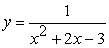is

(a)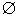(b)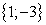(c) R \ {1} (d) R \ {1;-3}

The mathematical concept hides behind this question is “quadratic equation”. Whenever students recognize this underlying concept, the quadratic equation is easily solved by using a familiar procedure. For this question, we arrange it in the CK1 level. However, as we explain, this question can be considered as in the PK1 or PCK level.

On the other hand, if students use the property “A fraction is well-defined if its denominator is non-zero”, they can go directly to the procedure “Solving a quadratic equation”. This is an adaptation between the PK1 level in the PCK taxonomy to the dimension Properties in the SPUR multi-dimensional approach (denoted by PK1-P).

Now we are going to answer the second research question. The number of questions or part questions corresponding to each category of the SPUR multi-dimensional approach is presented in Table 7.

From this table, we have the following observations.

Ÿ The dimension Properties is assessed most frequently, especially with more than 48% at three schools, while the dimensions Uses and Representations are less appeared. One of the main reasons is that there are no realistic problems in the assessments, hence the category Uses appeared just as application of the considered concepts to other concepts.

Ÿ The same disadvantage appeared as in the PCK taxonomy, the distinction between dimensions Skills and Properties is not clear to recognize, because for some tasks, firstly students need to recognize which properties used to solve the problems, and then they use the known procedures to proceed solving them. By this process, we consider these tasks in the dimension Properties. For example, let us consider the following multiple-choice task.

The function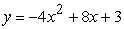is increasing monotone on which intervals

(a)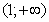(b)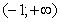(c)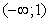(d)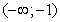?”

For this question, firstly students use the following property of the monotonicy of quadratic functions: “For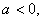the function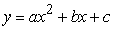is increasing monotone on the interval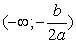.” Then they proceed by determining the coefficients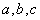of the given function and replace into the formula. By this way, we consider this task in the dimension Properties. However, we may think that the monotonic property given above of quadratic functions is a known procedure to find the increasing intervals, then students can go directly to look for the coefficientsand replace them into the formula. By this approach, we may consider this task as in the dimension Skills. This question can be considered as in the adaptation PK1-P.

On the other hand, students can draw the shape of the quadratic functionswhenbased on the drawn shape, they can determine the interval for which this function is increasing monotone. This is actually an adaptation of the dimension Representations to the level PK1, which is denoted by PK1-R.

The disadvantages mentioned above show that we need to have an adaptation of the PCK taxonomy to the multi-dimenisonal approach SPUR in the assessments of mathematical understanding. The number of questions or part questions corresponding to each category of the adaptation PCK to SPUR is presented in Table 8.

We have the following observation deduced from Table 8.

Ÿ For the adaptation PCK-Skills, the most frequently category assessed at three schools is CK1-S, with more than 42% questions or parts of questions assess fluent skill in recognizing the underlying concepts in solving problems.

Ÿ For the adaptation PCK-Properties, the most popular category assessed is PK1-P, with more than 48% questions or parts of questions focus on the recognition of which properties are used and applying familiar procedures and rules to solve the given problems.

Ÿ For the adaptation PCK-Uses, the category CK2-U appears more frequently, with more than 27% questions or parts of questions concentrate to assess the ability of students in applying the considered concept to other concepts. However, there are no questions or parts of questions at QHH assessing for the category PK1-U, and no questions at TVH and TCVH assessing for the category PK2-U. One of the main reasons is that there are no realistic problems in the assessments at three schools to use the familiar (resp. the complicated) procedures in solving the given tasks.

Ÿ For the adaptation PCK-Representation, in general there are not many questions assessing the adapted categories. Especially, there are no questions or parts of questions assessing the categories PK1-R and PK2-R. The most popular category appears in the assessments is CK1-R, with more than 16% questions or parts of questions focus on that.

### 3. Conclusion

Each written assessment is designed to meet four levels of the criteria for knowledge and skills applying now in Vietnam (Recognizing, Understanding, Applying (low level) and Applying (high level)). However, these levels are quite general to establish written assessments. The SPUR multi-dimensional approach (resp. the PCK taxonomy) gives more specialized dimensions (resp. levels) for the assessment establishment. However, there are some disadvantage while considering which tasks belong to which dimensions or levels. Therefore, an adaptation of the SPUR multi-dimensional approach to the PCK taxonomy is necessary, solving obstacles appear.

Since there are no realistic problems in the written assessments given at three high schools, the dimension Use in the SPUR approach (and its adaptation to five levels of the PCK taxonomy) is in general missed. Hence, in establishing the written assessments, it is necessary to study the content of each adapted category in the adaptation PCK-SPUR to distribute the tasks. On the other hand, including more realistic problems in the written assessments will make all categories in the adaptation PCK-SPUR fulfilled. Moreover, the adaptation of the CK2 level to four dimensions of SPUR will increase its appearance whenever the tasks in the assessments are established with open-ended questions.

### Acknowledgements

The author would like to express her deep gratitude to Dr. Tran Vui (Hue University’s College of Education, Vietnam), for his suggestion and helpful discussion to propose the new cognitive taxonomy PCK.

### References

  Thompson, D. R. and Senk, S. L., 2008, A multi-dimensional approach to understanding in mathematics textbooks developed by UCSMP. Paper presented in Discussion Group 17 of the International Congress on Mathematics Education. Monterrey, Mexico. In article  Thompson, D. R. and Kaur, B., 2011, Using a multi-dimensional approach to understanding to assess students’ mathematical knowledge. In B. Kaur & K. Y. Wong (Eds.), Assessment in the mathematics classroom, (pp. 17-32). Singapore: World Scientific Publishing. In article View Article  Bleiler, S.K. and Thompson, D.R., 2013, “Multidimensional assessment of CCSSM,” Teaching Children Mathematics, 19(5), 292-300. In article View Article  Wong, L. F., & Kaur, B., 2015, “A study of mathematics written assessment in Singapore secondary schools”, The Mathematics Educator, 16(1), 1-26. In article  Khaw, A. H. R. and Kaur, B., 2017, “A study of mathematics homework in Singapore Secondary Two classrooms,” The Mathematics Educator, 17(1), 29-56. In article  Hiebert, J. and Lefevre, P., 1986, Conceptual and procedural knowledge in mathematics: An introductory analysis. In J. Hiebert (Ed.), Conceptual and procedural knowledge: The case of mathematics (pp. 1-27). Hillsdale, NJ: Lawrence Erlbaum Associates. In article  Baroody, A. J. and Ginsburg, H. P., 1986, The relationship between initial meaningful and mechanical knowledge of arithmetic. In J. Hiebert (Ed.), Conceptual and procedural knowledge: The case of mathematics (pp. 75-112). Hillsdale, NJ, US: Lawrence Erlbaum Associates, Inc. In article  Rittle-Johnson, B. and Siegler, R. S., 1998, The relation between conceptual and procedural knowledge in learning mathematics: A review. In C. Donlan (Ed.), The development of mathematical skills (pp. 75-328). Hove, UK: Psychology Press. In article  Rittle-Johnson, B. and Alibali, R.M., 1999, Conceptualand procedural knowledge of mathematics: Does one lead to another? Journal of Educational Psychology, 91, 175-189. In article View Article  Barr, C., Doyle, J. M. C., Teresa D. L. and Carol D., 2003, “There is More to Math: A Framework for Learning and Math Instruction”, Waterloo Catholic District School Board. In article  Arslan, S., 2010, “Traditional instruction of differential equations and conceptual learning,” Teaching Mathematics and its Applications, 29 (2), 94-107. In article View Article  Rittle-Johnson, B. and Schneider, M., 2015, Developing conceptual and procedural knowledge in mathematics. In R. Cohen Kadosh and A. Dowker (Eds), Oxford handbook of numerical cognition, 1102-1118, UK: Oxford University Press. In article PubMed  Piper, B., Ralaingita, W., Akach, L. and King, S., 2016, “Improving procedural and conceptual mathematics outcomes: evidence from a randomised controlled trial in Kenya,” Journal of Development Effectiveness, 8, (3), 404-422. In article View Article  Bloom, B. S., Engelhart, M. D., Furst, E. J., Hill, W. H. and Krathwohl, D. R., 1956, Taxonomy of educational objectives: Handbook I: Cognitive domain. New York: David McKay. In article  Skemp, R., 1976, “Relational Understanding and Instrumental Understanding,” Mathematics Teaching, 77. 20-26. In article  Hiebert, J. and Carpenter, T. P., 1992, Learning and teaching with understanding. In D. A. Grouws (Ed.), Handbook of research on mathematics teaching and learning (pp. 65-97). New York: Mcmillan. In article  Barmby, P., Harries, T., Higgins, S. and Suggate, J., 2007, How can we assess mathematical understanding? In Woo, J. H., Lew, H. C., Park, K. S. and Seo, D. Y. (Eds.). Proceedings of the 31st Conference of the International Group for the Psychology of Mathematics Education, Vol. 2, 41-48. In article  Baroody, A. J., 2003, The Development of Adaptive Expertise and Flexibility: The Integration of Conceptual and Procedural Knowledge. In A. J. Baroody and A. Dowker (Eds.). The Development of Arithmetic Concepts and Skills, 1-34. In articleThis work is licensed under a Creative Commons Attribution 4.0 International License. To view a copy of this license, visit http://creativecommons.org/licenses/by/4.0/